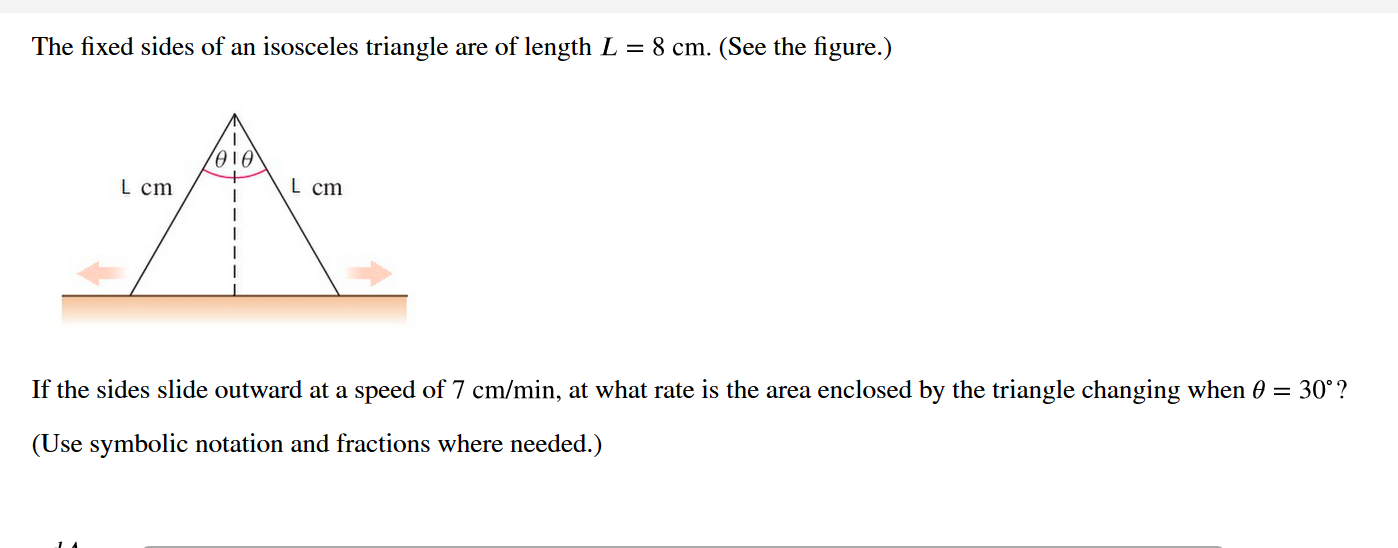# The fixed sides of an isosceles triangle are of length L = 8 cm. (See the figure.)L cmL cmIf the sides slide outward at a speed of 7 cm/min, at what rate is the area enclosed by the triangle changing when 0 = 30°?(Use symbolic notation and fractions where needed.)

Question
53 viewshelp_outlineImage TranscriptioncloseThe fixed sides of an isosceles triangle are of length L = 8 cm. (See the figure.) L cm L cm If the sides slide outward at a speed of 7 cm/min, at what rate is the area enclosed by the triangle changing when 0 = 30°? (Use symbolic notation and fractions where needed.) fullscreen
check_circle

star
star
star
star
star
1 Rating
Step 1

First of all, considering the half right-angled triangle whose area will be the same as the other half right-angled triangle. The isosceles triangle can be divided into two equal half right-angled triangle by the height of the triangle.

L = 8 cm, dx/dt = 7 cm/min

Step 2

Now, using the cosine formula get the value of h.

Step 3

Now, calculating the area of a right-angled triangle.

Then calculating the area for both the triangles to get the area of the total isosceles triangle.

The total area of the isosceles triangle will be twice the area of...

### Want to see the full answer?

See Solution

#### Want to see this answer and more?

Solutions are written by subject experts who are available 24/7. Questions are typically answered within 1 hour.*

See Solution
*Response times may vary by subject and question.
Tagged in

### Derivative